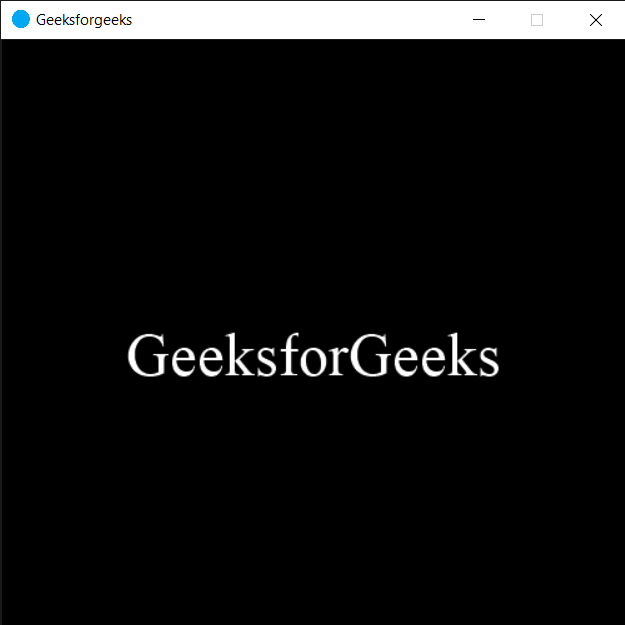Open In App

# PYGLET – On Text Motion Event

In this article we will see how we can trigger on text motion event in PYGLET module in python. Pyglet is easy to use but powerful library for developing visually rich GUI applications like games, multimedia etc. A window is a “heavyweight” object occupying operating system resources. Windows may appear as floating regions or can be set to fill an entire screen (fullscreen).Typically this is called after on_key_press() and before on_key_release(), but may also be called multiple times if the key is help down (key repeating). We should always use this method for moving the text input cursor (caret), as different platforms have different default keyboard mappings, and key repeats are handled correctly. We can create a window with the help of command given below

`pyglet.window.Window(width, height, title)`

Below is the syntax of the on text motion, this method get called when this event is triggered

```@window.event
def on_text_motion(motion):
print("Arrow Key pressed")```

Below are the list of motion this event covers MOTION_UP MOTION_RIGHT MOTION_DOWN MOTION_LEFT MOTION_NEXT_WORD MOTION_PREVIOUS_WORD MOTION_BEGINNING_OF_LINE MOTION_END_OF_LINE MOTION_NEXT_PAGE MOTION_PREVIOUS_PAGE MOTION_BEGINNING_OF_FILE MOTION_END_OF_FILE MOTION_BACKSPACE MOTION_DELETE

Below is the implementation

## Python3

 `# importing pyglet module``import` `pyglet``import` `pyglet.window.key` `# width of window``width ``=` `500` `# height of window``height ``=` `500` `# caption i.e title of the window``title ``=` `"Geeksforgeeks"` `# creating a window``window ``=` `pyglet.window.Window(width, height, title)` `# text``text ``=` `"GeeksforGeeks"` `# creating a label with font = times roman``# font size = 36``# aligning it to the center``label ``=` `pyglet.text.Label(text,``                          ``font_name ``=``'Times New Roman'``,``                          ``font_size ``=` `36``,``                          ``x ``=` `window.width``/``/``2``, y ``=` `window.height``/``/``2``,``                          ``anchor_x ``=``'center'``, anchor_y ``=``'center'``)` `new_label ``=` `pyglet.text.Label(text,``                          ``font_name ``=``'Times New Roman'``,``                          ``font_size ``=` `10``,``                          ``x ``=` `25``, y ``=` `25``)` `# on draw event``@window``.event``def` `on_draw():   ` `    ` `    ``# clearing the window``    ``window.clear()``    ` `    ``# drawing the label on the window``    ``label.draw()` `    ` `# key press event   ``@window``.event``def` `on_key_press(symbol, modifier):` `    ``# key "C" get press``    ``if` `symbol ``=``=` `pyglet.window.key.C:``        ` `        ``print``("Key C ``is` `pressed")`  `# on text motion event``@window``.event``def` `on_text_motion(motion):``    ` `    ``# printing message``    ``print``(motion)``    `  `# image for icon``img ``=` `image ``=` `pyglet.resource.image("logo.png")` `# setting image as icon``window.set_icon(img)` `               ` `# start running the application``pyglet.app.run()`

Output :When we press arrow key this message will get printed

```65362
65362
65362
65363
65363
65363
65361
65361
65361
65364```